Next: Electric Dipole Approximation Up: Time-Dependent Perturbation Theory Previous: Harmonic Perturbations

Let us use the above results to investigate the interaction of an atomic electron with classical (i.e., non-quantized) electromagnetic radiation.

The unperturbed Hamiltonian of the system is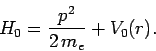(1080)

Now, the standard classical prescription for obtaining the Hamiltonian of a particle of chargein the presence of an electromagnetic field is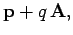(1081)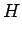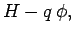(1082)

whereis the vector potential, andthe scalar potential. Note that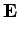(1083)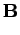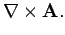(1084)

This prescription also works in quantum mechanics. Thus, the Hamiltonian of an atomic electron placed in an electromagnetic field is(1085)

where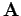and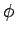are functions of the position operators. The above equation can be written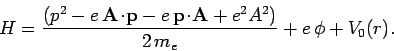(1086)

Now,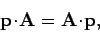(1087)

provided that we adopt the gauge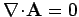. Hence,(1088)

Suppose that the perturbation corresponds to a linearly polarized, monochromatic, plane-wave. In this case,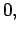(1089)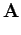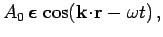(1090)

where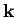is the wavevector (note that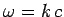), and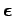a unit vector which specifies the direction of polarization (i.e., the direction of). Note that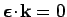. The Hamiltonian becomes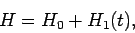(1091)

with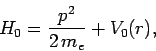(1092)

and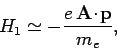(1093)

where the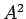term, which is second order in, has been neglected.

The perturbing Hamiltonian can be written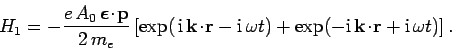(1094)

This has the same form as Eq. (1067), provided that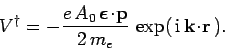(1095)

It follows from Eqs. (1069), (1079), and (1095) that the transition probability for radiation induced absorption is(1096)

Now, the mean energy density of an electromagnetic wave is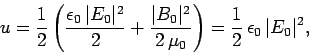(1097)

where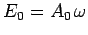and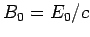are the peak electric and magnetic field-strengths, respectively. It thus follows that(1098)

Thus, not surprisingly, the transition probability for radiation induced absorption (or stimulated emission) is directly proportional to the energy density of the incident radiation.

Suppose that the incident radiation is not monochromatic, but instead extends over a range of frequencies. We can write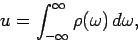(1099)

where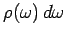is the energy density of radiation whose frequencies lie betweenand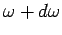. Equation (1098) generalizes to(1100)

Note, however, that the above expression is only valid provided the radiation in question is incoherent: i.e., there are no phase correlations between waves of different frequencies. This follows because it is permissible to add the intensities of incoherent radiation, whereas we must always add the amplitudes of coherent radiation. Given that the function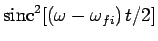is very strongly peaked (see Fig. 25) about(assuming that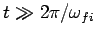), and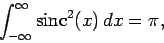(1101)

the above equation reduces to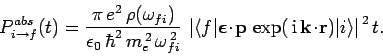(1102)

Note that in integrating over the frequencies of the incoherent radiation we have transformed a transition probability which is basically proportional to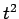[see Eq. (1098)] to one which is proportional to. As has already been explained, the above expression is only valid when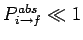. However, the result that(1103)

is constant in time is universally valid. Here,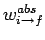is the transition probability per unit time interval, otherwise known as the transition rate. Given that the transition rate is constant, we can write (see Cha. 2)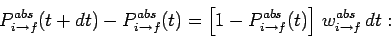(1104)

i.e., the probability that the system makes a transition from state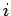to state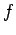between timesand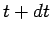is equivalent to the probability that the system does not make a transition between times 0 andand then makes a transition in a time interval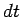--the probabilities of these two events are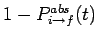and, respectively. It follows that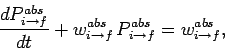(1105)

with the initial condition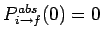. The above equation can be solved to give(1106)

This result is consistent with Eq. (1102) provided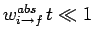: i.e., provided that.

Using similar arguments to the above, the transition probability for stimulated emission can be shown to take the form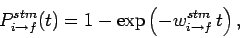(1107)

where the corresponding transition rate is written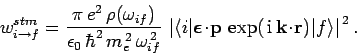(1108)Next: Electric Dipole Approximation Up: Time-Dependent Perturbation Theory Previous: Harmonic Perturbations
Richard Fitzpatrick 2010-07-20